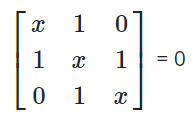# Mathematics 2020 Past Questions | JAMB

Study the following Mathematics past questions and answers for JAMBWAEC  NECO and Post-JAMB. Get prepared with official past questions and answers for upcoming examinations.

31. The chord ST of a circle is equal to the radius r of the circle. Find the length of arc ST

• A. πr/3
• B. πr/2
• C. πr/12
• D. πr/6

32. A sector of circle of radius 7.2cm which subtends an angle of 300oat the centre is used to form a cone. What s the radius of the base of the cone?

• A. 8cm
• B. 9cm
• C. 6cm
• D. 7cm

33. A cylindrical tank has a capacity of 3080m3. What is the depth of the tank if the diameter of its base is 14m?

• A. 25m
• B. 23m
• C. 22m
• D. 20m

34. The acres for rice, pineapple, cassava, cocoa and palm oil in a certain district are given respectively as 2, 5, 3, 11 and 9. What is the angle of the sector of cassava in a pie chart?

• A. 180o
• B. 36o
• C. 60o
• D. 108o

35. Three consecutive terms of a geometric progression are give as n – 2, n and n + 3. Find the common ratio

• A. 3/2
• B. 2/3
• C. 1/2
• D. 1/4

36. In a class of 40 students, 32 offer mathematics, 24 offer physics and 4 offer neither mathematics nor physics. How many offer both mathematics and physics?

• A. 4
• B. 8
• C. 16
• D. 20

37. The sum of the interior angle of pentagon is 6x + 6y. Find y in terms of x.

• A. y = 6 – x
• B. y = 90 – x
• C. y = 120 – x
• D. y = 150 – x

38. The mean age group of some students is 15years. When the age of a teacher, 45 years old, is added to the ages of the students, the mean of their ages become 18 years. Find the number of students in the group.

• A. 7
• B. 9
• C. 15
• D. 42

39. A surveyor walks 500m up a hill which slopes at an angle of 30o. Calculate the vertical height through which he rises

• A. 252m
• B. 500m
• C. 250m
• D. 255m

40. Find the non-zero positive value of x which satisfies the equation• A. 2
• B. √3
• C. √2
• D. 1

Scroll to Top